Refer to our Texas Go Math Grade 1 Answer Key Pdf to score good marks in the exams. Test yourself by practicing the problems from Texas Go Math Grade 1 Module 11 Assessment Answer Key.

Concepts and Skills

Use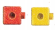. Complete the model and the number sentence.

Question 1.
6 bunnies sit in the grass. 2 more bunnies join them. How many bunnies are in the grass now? (TEKS 1.5.D)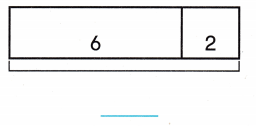6 + 2 = ___________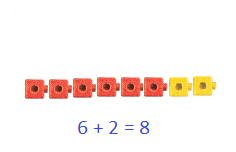Given,
There are six bunnies in the grass and two bunnies join them
By adding six with two we get eight,
So there are total of eight bunnies in the grass.
6 + 2 = 8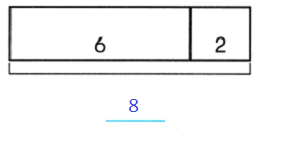Question 2.
There are 2 people in the house. Some more people go in the house. Now there are 6 people in the house. How many people go in the house? (TEKS 1.5.D)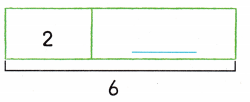2 + __________ = 6Given,
There are two people in the house, some people go into the house
Now there are total of 6 people in the house,
We know that,
By subtracting two from six we get four,
So four people went into the house.Question 3.
Jennifer has 3 pennies. Brad has 9 pennies. How many fewer pennies does Jennifer have than Brad? (TEKS 1.5.D)Given,
Jennifer has three pennies, Brad has nine pennies
By subtracting three from nine we get six.
So Jennifer has six number of pennies less than Brad.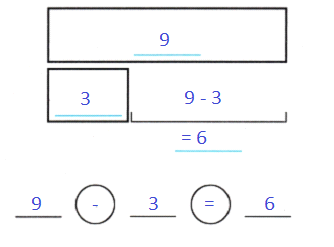Use. Choose the correct answer

Question 4.
There are 9 beads. 5 beads are pink. The rest are yellow. How many beads are yellow? (TEKS 1.5.D)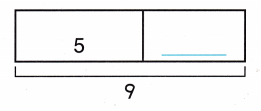(A) 5
(B) 9
(C) 4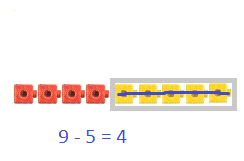Given,
There are a total of nine beads, five of them are pink beads and the rest are yellow beads
By subtracting five from nine we get four,
The correct answer is option C

Question 5.
Mandy has 2 rocks. Peter has 8 rocks. How many more rocks does Peter have than Mandy? (TEKS 1.5.D)(A) 8 – 2 = 6
(B) 6 – 2 = 4
(C) 8 – 3 = 5Given,
Mandy has two rocks, Peter has eight rocks
By subtracting two from eight we get six,
Peter has six more rocks than Mandy,
So the correct answer is option A.

Question 6.
Pat picks 18 flowers. 9 are yellow. The rest are pink. How many are pink? (TEKS 1.5.D)(A) 8
(B) 9
(C) 7